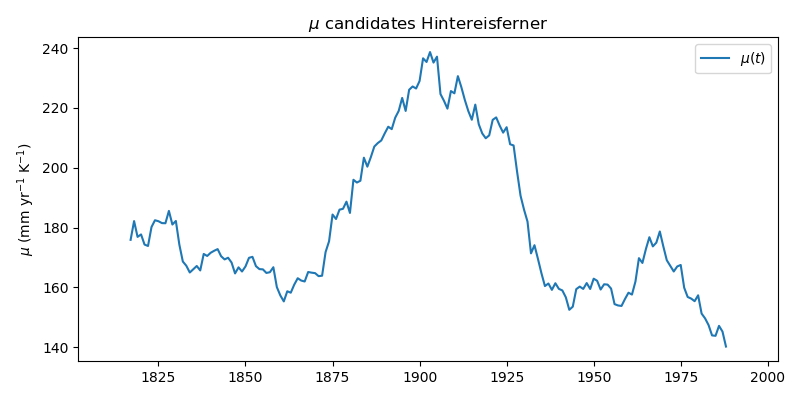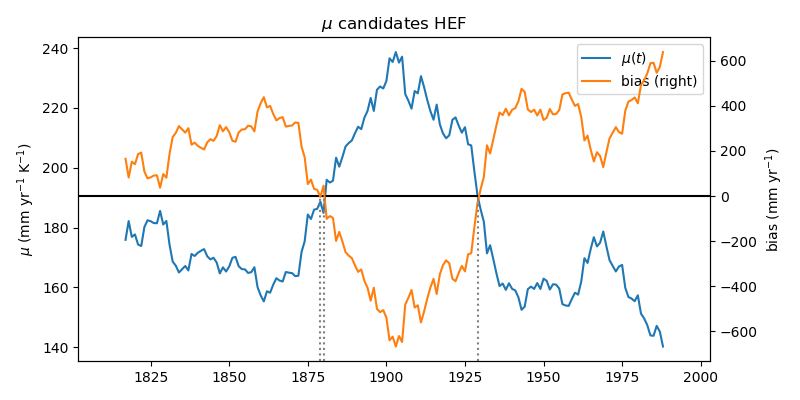# Mass-balance¶

The mass-balance (MB) model implemented in OGGM is an extended version of the temperature index model presented by Marzeion et al., (2012). While the equation governing the mass-balance is that of a traditional temperature index model, our special approach to calibration requires that we spend some time describing it.

## Climate data¶

The MB model implemented in OGGM needs monthly time series of temperature and precipitation. The current default is to download and use the CRU TS v3.24 data provided by the Climatic Research Unit of the University of East Anglia.

### CRU (default)¶

If not specified otherwise, OGGM will automatically download and unpack the latest dataset from the CRU servers.

Warning

While the downloaded zip files are ~370mb in size, they are ~5.6Gb large after decompression!

The raw, coarse (0.5°) dataset is then downscaled to a higher resolution grid (CRU CL v2.0 at 10’ resolution) following the anomaly mapping approach described by Tim Mitchell in his CRU faq (Q25). Note that we don’t expect this downscaling to add any new information than already available at the original resolution, but this allows us to have an elevation-dependent dataset, from which we can compute the temperature at the elevation of the glacier.

### User-provided dataset¶

You can provide any other dataset to OGGM by setting the climate_file parameter in params.cfg. See the HISTALP data file in the sample-data folder for an example.

In : example_plot_temp_ts()  # the code for these examples is posted below### Elevation dependency¶

OGGM finally needs to compute the temperature and precipitation at the altitude of the glacier grid points. The default is to use a fixed gradient of -6.5K km $$^{-1}$$ and no gradient for precipitation. However, OGGM implements a module which computes the local gradient by linear regression of the 9 surrounding grid points. This method requires that the near-surface temperature lapse-rates provided by the climate dataset are good (in most of the cases you should probably use a fixed gradient). The default config parameters are:

In : cfg.PARAMS['temp_use_local_gradient']  # use the regression method?
Out: False

Out: -0.0065


## Temperature index model¶

The monthly mass-balance $$B_i$$ at elevation $$z$$ is computed as:

$B_i(z) = P_i^{Solid}(z) - \mu ^{*} \, max \left( T_i(z) - T_{Melt}, 0 \right)$

where $$P_i^{Solid}$$ is the monthly solid precipitation, $$T_i$$ the monthly temperature and $$T_{Melt}$$ is the monthly mean air temperature above which ice melt is assumed to occur (0°C per default). Solid precipitation is computed out of the total precipitation. The fraction of solid precipitation is based on the monthly mean temperature: all solid below temp_all_solid (default: 0°C) all liquid above temp_all_liq (default: 2°C), linear in between.

The parameter $$\mu ^{*}$$ indicates the temperature sensitivity of the glacier, and it needs to be calibrated.

## Calibration¶

We will start by making two observations:

• the sensitivity parameter $$\mu ^{*}$$ is depending on many parameters, most of them being glacier-specific (e.g. avalanches, topographical shading, cloudiness…).
• the sensitivity parameter $$\mu ^{*}$$ will be affected by uncertainties and systematic biases in the input climate data.

As a result, $$\mu ^{*}$$ can vary greatly between neighboring glaciers. The calibration procedure introduced by Marzeion et al., (2012) and implemented in OGGM makes full use of these apparent handicaps by turning them into assets.

The calibration procedure starts with glaciers for which we have direct observations of the annual specific mass-balance SMB. We use the WGMS FoG (shipped with OGGM) for this purpose.

For each of these glaciers, time-dependent “candidate” temperature sensitivities $$\mu (t)$$ are estimated by requiring that the average specific mass-balance $$B_{31}$$ is equal to zero. $$B_{31}$$ is computed for a 31 yr period centered around the year $$t$$ and for a constant glacier geometry fixed at the RGI date (e.g. 2003 for most glaciers in the European Alps).

In : example_plot_mu_ts()  # the code for these examples is posted belowAround 1900, the climate was cold and wet. As a consequence, the temperature sensitivity required to maintain the 2003 glacier geometry is high. Inversely, the recent climate is warm and the glacier must have a small temperature sensitivity in order to preserve its geometry.

Note that these $$\mu (t)$$ are just hypothetical sensitivities necessary to maintain the glacier in equilibrium in an average climate at the year $$t$$. We call them “candidates”, since one (or more) of them is likely to be close to the “real” sensitivity of the glacier.

This is when the mass-balance observations come into play: each of these candidates can be used to compute the mass-balance during the period were we have observations. We then compare the model output with the expected mass-balance and compute the model bias:

In : example_plot_bias_ts()  # the code for these examples is posted belowThe bias is positive when $$\mu$$ is too low, and negative when $$\mu$$ is too high. The vertical dashed lines mark the times where the bias is closest to zero. They all correspond to approximately the same $$\mu$$ (but not exactly, as precipitation and temperature both influence $$\mu$$). These dates at which the $$\mu$$ candidates are close to the real $$\mu$$ are called $$t^*$$ (the associated sensitivities $$\mu (t^*)$$ are called $$\mu^*$$).

At the glaciers where observations are available, this detour via the $$\mu$$ candidates is not necessary to find the correct $$\mu^*$$. Indeed, the goal of these computations are in fact to find $$t^*$$, which is the actual value to be interpolated to glaciers where no observations are available.

The benefit of this approach is best shown with the results of a cross-validation study realized by Marzeion et al., (2012) (and confirmed by OGGM):Benefit of spatially interpolating $$t^{*}$$ instead of $$\mu ^{*}$$ as shown by leave-one-glacier-out cross-validation (N = 255). Left: error distribution of the computed mass-balance if determined by the interpolated $$t^{*}$$. Right: error distribution of the mass-balance if determined by interpolation of $$\mu ^{*}$$.

This substantial improvement in model performance is due to several factors:

• the equilibrium constraint applied on $$\mu$$ implies that the sensitivity cannot vary much during the last century. In fact, $$\mu$$ at one glacier varies far less in one century than between neighboring glaciers, because of all the factors mentioned above. In particular, it will vary comparatively little around a given year $$t$$ : errors in $$t^*$$ (even large) will result in small errors in $$\mu^*$$.
• the equilibrium constraint will also imply that systematic biases in temperature and precipitation (no matter how large) will automatically be compensated by all $$\mu (t)$$, and therefore also by $$\mu^*$$. In that sense, the calibration procedure can be seen as a empirically driven downscaling strategy: if a glacier is here, then the local climate (or the glacier temperature sensitivity) must allow a glacier to be there. For example, the effect of avalanches or a negative bias in precipitation input will have the same impact on calibration: $$\mu^*$$ should be reduced to take these effects into account, even though they are not resolved by the mass-balance model.

The most important drawback of this calibration method is that it assumes that two neighboring glaciers should have a similar $$t^*$$. This is not necessarily the case, as other factors than climate (such as the glacier size) will influence $$t^*$$ too. Our results (and the arguments listed above) show however that this is an approximation we can cope with.

In a final note, it is important to mention that the $$\mu^*$$ and $$t^*$$ should not be over-interpreted in terms of “real” temperature sensitivities or “real” response time of the glacier. This procedure is primarily a calibration method, and as such it can be statistically scrutinized (for example with cross-validation). It can also be noted that the MB observations play a relatively minor role in the calibration: they could be entirely avoided by fixing a $$t^*$$ for all glaciers in a region (or even worldwide). The resulting changes in calibrated $$\mu^*$$ will be comparatively small (again, because of the local constraints on $$\mu$$). The MB observations, however, play a major role for the assessment of model uncertainty.

## Implementation details¶

If you had the courage to read until here, it means that you have concrete questions about the implementation of the mass-balance model in OGGM. Here are some more details:

• the mass-balance in OGGM is computed from the altitudes and widths of the flowlines grid points (see Glacier flowlines). The easiest way to let OGGM compute the mass-balance for you is to use the core.massbalance.PastMassBalance.
• the interpolation of $$t^*$$ is done with an inverse distance weighting algorithm (see tasks.distribute_t_stars())
• if more than one $$t^*$$ is found for some reference glaciers, than the glaciers with only one $$t^*$$ will determine the most likely $$t^*$$ for the other glaciers (see tasks.compute_ref_t_stars())
• yes, the temperature gradients and the precipitation scaling factor will have an influence on the results, but it is small since any change will automatically be compensated by $$\mu^*$$. We are currently quantifying these effects more precisely.
• the cross-validation procedure is done systematically (tasks.crossval_t_stars()). Currently, this cross-validation is done with fixed geometry (it will be extended to a full validation with the dynamical model at a later stage).

Code used to generate these examples:

import os

import geopandas as gpd
import matplotlib.pyplot as plt
import numpy as np
import pandas as pd
import xarray as xr

import oggm
from oggm.core.climate import (mb_yearly_climate_on_glacier,
t_star_from_refmb,
local_mustar, apparent_mb)
from oggm.core.massbalance import (ConstantMassBalance)
from oggm.utils import get_demo_file
from oggm import graphics

cfg.initialize()
cfg.set_intersects_db(get_demo_file('rgi_intersect_oetztal.shp'))
cfg.PATHS['dem_file'] = get_demo_file('hef_srtm.tif')
pcp_fac = 2.6
cfg.PARAMS['prcp_scaling_factor'] = pcp_fac

base_dir = os.path.join(os.path.expanduser('~'), 'Climate')
gdir = oggm.GlacierDirectory(entity, base_dir=base_dir)

cfg.PATHS['climate_file'] = get_demo_file('histalp_merged_hef.nc')

mbdf = gdir.get_ref_mb_data()
res = t_star_from_refmb(gdir, mbdf.ANNUAL_BALANCE)
local_mustar(gdir, tstar=res['t_star'][-1], bias=res['bias'][-1],
prcp_fac=res['prcp_fac'], reset=True)
apparent_mb(gdir, reset=True)

# For flux plot

# For plots
years, temp_yr, prcp_yr = mb_yearly_climate_on_glacier(gdir, pcp_fac)

# which years to look at
selind = np.searchsorted(years, mbdf.index)
temp_yr = np.mean(temp_yr[selind])
prcp_yr = np.mean(prcp_yr[selind])

# Average oberved mass-balance
ref_mb = mbdf.ANNUAL_BALANCE.mean()
mb_per_mu = prcp_yr - mu_yr_clim * temp_yr

# Diff to reference
diff = mb_per_mu - ref_mb
pdf = pd.DataFrame()
pdf[r'$\mu (t)$'] = mu_yr_clim
pdf['bias'] = diff
res = t_star_from_refmb(gdir, mbdf.ANNUAL_BALANCE)

# For the mass flux
mbmod = ConstantMassBalance(gdir)
mbx = mbmod.get_annual_mb(cl['hgt']) * cfg.SEC_IN_YEAR * cfg.RHO
fdf = pd.DataFrame(index=np.arange(len(mbx))*cl['dx'])
fdf['Flux'] = cl['flux']
fdf['Mass balance'] = mbx

# For the distributed thickness

# plot functions
def example_plot_temp_ts():
d = xr.open_dataset(gdir.get_filepath('climate_monthly'))
temp = d.temp.resample(freq='12MS', dim='time', how=np.mean).to_series()
del temp.index.name
ax = temp.plot(figsize=(8, 4), label='Annual temp')
temp.rolling(31, center=True, min_periods=15).mean().plot(label='31-yr avg')
plt.legend(loc='best')
plt.title('HISTALP annual temperature, Hintereisferner')
plt.ylabel(r'degC')
plt.tight_layout()
plt.show()

def example_plot_mu_ts():
ax = mu_yr_clim.plot(figsize=(8, 4), label=r'$\mu (t)$');
plt.legend(loc='best'); plt.title(r'$\mu$ candidates Hintereisferner');
plt.ylabel(r'$\mu$ (mm yr$^{-1}$ K$^{-1}$)')
plt.tight_layout()
plt.show()

def example_plot_bias_ts():
ax = pdf.plot(figsize=(8, 4), secondary_y='bias')
plt.hlines(0, 1800, 2015, linestyles='-')
ax.set_ylabel(r'$\mu$ (mm yr$^{-1}$ K$^{-1}$)');
ax.set_title(r'$\mu$ candidates HEF');
plt.ylabel(r'bias (mm yr$^{-1}$)')
yl = plt.gca().get_ylim()
for ts in res['t_star']:
plt.plot((ts, ts), (yl, 0), linestyle=':', color='grey')
plt.ylim(yl)
plt.tight_layout()
plt.show()

def example_plot_massflux():
fig, ax = plt.subplots(figsize=(8, 4))
fdf.plot(ax=ax, secondary_y='Mass balance', style=['C1-', 'C0-'])
plt.axhline(0., color='grey', linestyle=':')
ax.set_ylabel('Flux [m$^3$ s$^{-1}$]')
ax.right_ax.set_ylabel('MB [kg m$^{-2}$ yr$^{-1}$]')
ax.set_xlabel('Distance along flowline (m)')
plt.title('Mass flux and mass balance along flowline')
plt.tight_layout()
plt.show()